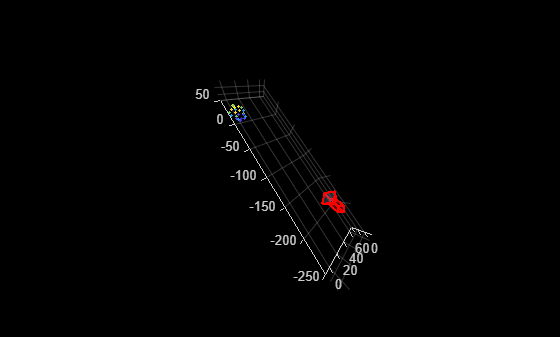# estworldpose

Estimate camera pose from 3-D to 2-D point correspondences

Since R2022b

## Syntax

``````worldPose = estworldpose(imagePoints,worldPoints,intrinsics)``````
``````[worldPose,inlierIdx] = estworldpose(imagePoints,worldPoints,intrinsics)``````
``[worldPose,inlierIdx,status] = estworldpose(imagePoints,worldPoints,intrinsics)``
``[___] = estworldpose(___,Name=Value)``

## Description

example

``````worldPose = estworldpose(imagePoints,worldPoints,intrinsics)``` returns the pose of a calibrated camera in a world coordinate system. The input `worldPoints` must be defined in the world coordinate system.This function solves the perspective-n-point (PnP) problem using the perspective-three-point (P3P) algorithm . The function eliminates spurious outlier correspondences using the M-estimator sample consensus (MSAC) algorithm. The inliers are the correspondences between image points and world points that are used to compute the camera pose.```
``````[worldPose,inlierIdx] = estworldpose(imagePoints,worldPoints,intrinsics)``` returns the indices of the inliers used to compute the camera pose, in addition to the arguments from the previous syntax.```
````[worldPose,inlierIdx,status] = estworldpose(imagePoints,worldPoints,intrinsics)` additionally returns a status code to indicate whether there were enough points.```
````[___] = estworldpose(___,Name=Value)` uses additional options specified by one or more name-value arguments, using any of the preceding syntaxes.```

## Examples

collapse all

```load("worldToImageCorrespondences.mat"); cameraParams.ImageSize = [128 128]; intrinsics = cameraParams.Intrinsics;```

Estimate the world camera pose.

`worldPose = estworldpose(imagePoints,worldPoints,intrinsics);`

Plot the world points.

```pcshow(worldPoints,VerticalAxis="Y",VerticalAxisDir="down", ... MarkerSize=30); hold on plotCamera(Size=10,Orientation=worldPose.R', ... Location=worldPose.Translation); hold off```## Input Arguments

collapse all

Coordinates of undistorted image points, specified as an M-by-2 array of [x, y] coordinates. The number of image points, M, must be at least four.

The function does not account for lens distortion. You can either undistort the images using the `undistortImage` function before detecting the image points, or you can undistort the image points themselves using the `undistortPoints` function.

Data Types: `single` | `double`

Coordinates of world points, specified as an M-by-3 array of [x, y, z] coordinates.

Data Types: `single` | `double`

Camera intrinsics, specified as a `cameraIntrinsics` object.

### Name-Value Arguments

Specify optional pairs of arguments as `Name1=Value1,...,NameN=ValueN`, where `Name` is the argument name and `Value` is the corresponding value. Name-value arguments must appear after other arguments, but the order of the pairs does not matter.

Example: `MaxNumTrials=1000`

Maximum number of random trials, specified as a positive integer scalar. The actual number of trials depends on the number of image and world points, and the values for the `MaxReprojectionError` and `Confidence` properties. Increasing the number of trials improves the robustness of the output at the expense of additional computation.

Confidence for finding maximum number of inliers, specified as a scalar in the range (0, 100). Increasing this value improves the robustness of the output at the expense of additional computation.

Reprojection error threshold for finding outliers, specified as a positive numeric scalar in pixels. Increasing this value makes the algorithm converge faster, but can reduce the accuracy of the result. Correspondences with a reprojection error larger than the `MaxReprojectionError` are considered outliers, and are not used to compute the camera pose.

## Output Arguments

collapse all

Camera pose in world coordinates, returned as a `rigidtform3d` object. The `R` and the `Translation` properties of the object represent the orientation and location of the camera.

Indices of inlier points, returned as an M-by-1 logical vector. A logical true value in the vector corresponds to inliers represented in `imagePoints` and `worldPoints`.

Status code, returned as `0`, `1`, or `2`.

Status codeStatus
`0`No error
`1``imagePoints` and `worldPoints` do not contain enough points. A minimum of four points are required.
`2`Not enough inliers found. A minimum of 4 inliers are required.

## Tips

 Gao, X.-S., X.-R. Hou, J. Tang, and H.F. Cheng. "Complete Solution Classification for the Perspective-Three-Point Problem." IEEE Transactions on Pattern Analysis and Machine Intelligence. Volume 25,Issue 8, pp. 930–943, August 2003.

 Torr, P.H.S., and A. Zisserman. "MLESAC: A New Robust Estimator with Application to Estimating Image Geometry." Computer Vision and Image Understanding. 78, no. 1 (April 2000): 138–56. https://doi.org/10.1006/cviu.1999.0832.